# Insert 7

Insert five harmonic means between 3 and 18

a2 =  3.4839
a3 =  4.1538
a4 =  5.1429
a5 =  6.75
a6 =  9.8182

### Step-by-step explanation: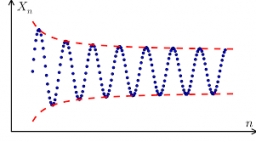Did you find an error or inaccuracy? Feel free to write us. Thank you!Tips to related online calculators
Looking for calculator of harmonic mean?
Looking for a statistical calculator?

## Related math problems and questions:

• Insert 6Insert four harmonic means between 3/7 and 3/19
• Harmonic seriesInsert four members between 5/3 and 5/11 to form harmonic series (means).
• Insert 5Insert five harmonic means between 1/2 and 1/26
• Harmonic mean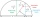If x, y, z form a harmonic progression, then y is the harmonic mean of x and z. Find the harmonic mean of the numbers 6 and 5.
• Insert 3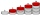Insert five arithmetic progression members between -7 and 3/2.
• HP - harmonic progression 2Compute the 16th term of the HP if the 6th and 11th term of the harmonic progression are 10 and 18 respectively.
• HP - harmonic progressionDetermine the 10th term of the harmonic progression 6,4,3,…
• Harmonic meanHarmonic means of 6 and 12
• HP - harmonic progressionDetermine the 8th term of the harmonic progression 2, 4/3, 1,…
• Insert AP memberInsert arithmetic means between 75 and 180.
• Insert 4Insert three arithmetic means between 3 and 63.
• Harmonic 4The harmonic mean of -6 and 5.
• PoolIf water flows into the pool by two inlets, fill the whole for 19 hours. The first inlet filled pool 5 hour longer than the second. How long pool take to fill with two inlets separately?
• Sequence 11What is the nth term of this sequence 1, 1/2, 1/3, 1/4, 1/5?
• Three children3 children eat 8 chocolates in 6 days. How many chocolates 6 children eat in 18 days?
• Cleaners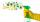Milan would clean up the room for 2.5 hours, Eric would take 10 hours. How long they swept the room together?
• A report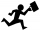Maricris can type a committee report in 5 hours. Jane helped Maricris and together they finished the report in 3 hours. How long would it take Jane to complete the report she had worked alone?